## find distance between (0,6) and (8,0)​ with process-…

Question

find distance between (0,6) and (8,0)​
with process…….

in progress 0
3 weeks 2021-08-30T20:53:39+00:00 2 Answers 0 views 0

answer to the question is 10 units..

10 units

Step-by-step explanation:

(0 , 6) = (x1 , y1)

(8 , 0) = (x2 , y2)

distance formula =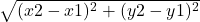=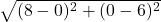=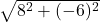=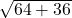=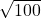=10 units